## Abstract

The aim of the present study is to investigate the Hall and ion slip currents on an incompressible free convective flow, heat and mass transfer of a micropolar fluid in a porous medium between expanding or contracting walls with chemical reaction, Soret and Dufour effects. Assume that the walls are moving with a time dependent rate of the distance and the fluid is injecting or sucking with an absolute velocity. The walls are maintained at constant but different temperatures and concentrations. The governing partial differential equations are reduced into nonlinear ordinary differential equations by similarity transformations and then the resultant equations are solved numerically by quasilinearization technique. The results are analyzed for velocity components, microrotation, temperature and concentration with respect to different fluid and geometric parameters and presented in the form of graphs. It is noticed that with the increase in chemical reaction, Hall and ion slip parameters the temperature of the fluid is enhanced whereas the concentration is decreased. Also for the Newtonian fluid, the numerical values of axial velocity are compared with the existing literature and are found to be in good agreement.

## Keywords

Micropolar fluid; Chemical reaction; Soret effect; Dufour effect; Hall and ion slip; Expanding or contracting walls

## Nomenclature

t- time

a(t)- distance between the origin and lower/upper wall

${\textstyle V_{1}}$- injection/suction velocity

p- fluid pressure

${\textstyle {\overline {q}}}$- velocity vector

c- specific heat at constant temperature

${\textstyle {\overline {l}}}$- microrotation vector

N- microrotation component

Ec- Eckert number, ${\textstyle {\frac {\mu V_{1}}{\rho ac(T_{2}-T_{1})}}}$

k- thermal conductivity

k1- viscosity parameter

k2- permeability of the medium

k3- chemical reaction rate

u- velocity component in x-direction

v- velocity component in y-direction

Pr- Prandtl number, ${\textstyle {\frac {\mu c}{k}}}$

Re- suction/injection Reynolds number, ${\textstyle {\frac {\rho V_{1}a}{\mu }}}$

j- gyration parameter

${\textstyle {\overline {J}}}$- current density

${\textstyle J_{1}}$- nondimensional gyration parameter, ${\textstyle {\frac {\rho {jaV}_{1}}{\gamma }}}$

${\textstyle {\overline {B}}}$- total magnetic field

${\textstyle {\overline {b}}}$- induced magnetic field

${\textstyle B_{0}}$- magnetic flux density

D- rate of deformation tensor

${\textstyle {\overline {E}}}$- electric field

Ha- Hartmann number, ${\textstyle B_{0}a{\sqrt {\frac {\sigma }{\mu }}}}$

D−1- inverse Darcy parameter, ${\textstyle {\frac {a^{2}}{k_{2}}}}$

R- nondimensional viscosity parameter, ${\textstyle {\frac {k_{1}}{\mu }}}$

${\textstyle s_{1}}$- nondimensional micropolar parameter, ${\textstyle {\frac {k_{1}a^{2}}{\gamma }}}$

${\textstyle s_{2}}$- nondimensional micropolar parameter, ${\textstyle {\frac {\gamma c}{a^{2}k}}}$

T- temperature

${\textstyle T_{1}}$- temperature of the lower wall

${\textstyle T_{2}}$- temperature of the upper wall

${\textstyle T^{_{\ast }}}$- dimensionless temperature, ${\textstyle {\frac {T-T_{1}}{T_{2}-T_{1}}}}$

C- concentration

C- nondimensional concentration, ${\textstyle {\frac {C-C_{1}}{C_{2}-C_{1}}}}$

C1- concentration of the lower wall

C2- concentration of the upper wall

D1- mass diffusivity

Kr- nondimensional chemical reaction parameter, ${\textstyle {\frac {k_{3}a^{2}}{D_{1}}}}$

Sc- Schmidt number, ${\textstyle {\frac {\upsilon }{D_{1}}}}$

Gr- thermal Grashof number, ${\textstyle {\frac {\rho g{\beta }_{T}(T_{2}-T_{1})a^{3}}{{\upsilon }^{2}}}}$

Gc- solutal Grashof number, ${\textstyle {\frac {\rho g{\beta }_{C}(C_{2}-C_{1})a^{3}}{{\upsilon }^{2}}}}$

Sh- Sherwood number, ${\textstyle {\frac {\overset {\cdot }{n_{A}}}{a\upsilon \left(C_{2}-C_{1}\right)}}}$

${\textstyle {\overset {\cdot }{n_{A}}}}$- mass transfer rate

Sr- Soret number, ${\textstyle {\frac {D_{1}k_{T}\upsilon V_{1}}{{cT}_{m}{\overset {\cdot }{n_{A}}}}}}$

Du- Dufour number, ${\textstyle {\frac {D_{1}k_{T}{\overset {\cdot }{n_{A}\rho c}}}{{\upsilon }^{2}V_{1}c_{s}k}}}$

${\textstyle T_{m}}$- mean temperature

${\textstyle k_{T}}$- thermal-diffusion ratio

${\textstyle c_{s}}$- concentration susceptibility

### Greek letters

${\textstyle \lambda }$- dimensionless y   coordinate, ${\textstyle {\frac {y}{a}}}$

${\textstyle \alpha {\mbox{,}}\beta {\mbox{,}}\gamma }$- gyro viscosity parameters

${\textstyle \zeta }$- dimensionless axial variable, ${\textstyle {\frac {x}{a}}}$

${\textstyle \upsilon }$- kinematic viscosity

${\textstyle \rho }$- fluid density

${\textstyle \mu }$- fluid viscosity

${\textstyle {\mu }^{'}}$- magnetic permeability

${\textstyle \sigma }$- conductivity

${\textstyle \eta }$- wall expansion ratio, ${\textstyle {\frac {a{\overset {\cdot }{a}}}{\upsilon }}}$

## 1. Introduction

The flow through porous channels has many important applications, in both engineering and biophysical flows. Examples of this include cosmetic industry, petroleum industry, soil mechanics, food preservation, the mechanics of the cochlea in the human ear, blood flow and artificial dialysis. Many researchers have investigated the numerical simulation of blood flow through curved geometries in the presence of a magnetic field by considering the effect of Dean number  and . The theory of micropolar fluids was introduced by Eringen  which are considered as an extension of generalized viscous fluids with microstructure. Examples of micropolar fluids include lubricants, colloidal suspensions, porous rocks, aerogels, polymer blends, micro emulsions. Micropolar fluid flow in a porous channel was studied by Ashraf et al.  and drawn numerical solution using the finite difference scheme. Ojjela and Naresh Kumar  obtained a numerical solution by the quasilinearization method for the MHD flow and heat transfer of a micropolar fluid through a porous channel. Chamkha et al.  considered the transient free convective-radiative micropolar fluid flow over the vertical porous plate with the chemical reaction and Joule heating. Aurangzaib et al.  investigated the problem of thermophoresis effect on MHD micropolar fluid flow over a stretching surface with Soret and Dufour effects. Srinivasacharya and RamReddy  examined the steady convective flow of a micropolar fluid over a vertical plate in a non-Darcian porous medium with Soret and Dufour effects and a numerical solution was obtained by Keller-Box method. The MHD flow of micropolar fluid through concentric cylinders with the chemical reaction and cross diffusion effects was studied by Srinivasacharya and Shiferaw . The Magnetohydrodynamic flow of a micropolar fluid with Hall and ion slip currents plays a great significance role in the real world applications in engineering. Ayano  considered the mixed convective micropolar fluid flow with heat and mass transfer in the presence of Hall and ion slip currents and the reduced governing equations are solved by the Keller-box method. The MHD flow of a micropolar fluid over a vertical plate with Hall and ion slip currents was investigated numerically by Anika et al. . An analytical approximate solution HAM is applied to the effect of space porosity on mixed convection flow of micropolar fluid through a vertical channel with double diffusion and viscous dissipation was investigated by Muthuraj et al. . Vedavathi et al.  illustrated the Soret and Dufour effects on the free convective flow of a viscous fluid past a vertical plate with radiation. The effects of Hall and ion slip on the mixed convection heat and mass transfer of second grade fluid with Soret and Dufour effects were investigated analytically by Hayat and Nawaz . Chamkha and Ben-Nakhi  considered the mixed convection flow of a radiating viscous fluid along a permeable surface in a porous medium with Soret and Dufour effects. Soret and Dufour effects on free convective heat and mass transfer of incompressible viscous fluid from a vertical cone in a saturated porous medium with varying wall temperature and concentration were studied by Cheng . The MHD flow of a viscous fluid over a vertical porous plate with Hall current has been considered by Anika et al. .

The study of magnetohydrodynamic flow, heat and mass transfer through porous expanding or contracting channels is attracted by many authors due to great applications in science and technology, such as transport of biological fluids through contracting or expanding vessels, the synchronous pulsating of porous diaphragms, the expanding or contracting jets, transpiration cooling and gaseous diffusion, the air circulation in the respiratory system, boundary layer control, and MHD pumps. Ojjela and Naresh Kumar  studied numerically the flow and heat transfer of chemically reacting micropolar fluid in porous expanding or contracting walls with slip velocity and Hall and ion slip effects. Si et al.  and  analyzed the problems of flow and heat transfer of micropolar fluids with expanding or contracting walls and obtained analytical solution by the Homotopy analysis method. The two dimensional unsteady viscous fluid flow between expanding or contracting walls with permeability investigated by Majdalani et al.  and the problem was solved both numerically and analytically. The viscous fluid flow through expanding and contracting walls with a less permeability has been studied by Asghar et al.  and obtained an analytical solution by Adomain decomposition method. The same problem with Lie-group method was considered by Boutros et al. . Uchida and Akoi  analyzed the laminar incompressible flow of a viscous fluid through a semi-infinite porous pipe whose radius varied with time. The heat and mass transfer analysis for the laminar flow between expanding or contracting walls with thermal diffusion and diffusion effects was discussed by Subramanyam Reddy et al. . Hymavathi and Shanker  and Hymavathi  applied the quasilinearization method to solve the visco-elastic fluid flow and heat transfer through a nonisothermal stretching sheet. The convective flow and heat transfer of viscous fluid in a vertical channel was studied by Huang  and applied the quasilinearization method to solve the problem.

In the present study, the effects of Hall and ion slip on two dimensional free convection flow and heat transfer of chemically reacting micropolar fluid in a porous medium between expanding or contracting walls with Soret and Dufour are considered. The reduced flow field equations are solved using the quasilinearization method. The effects of various parameters such as inverse Darcy’s parameter, chemical reaction rate, Soret effect, Dufour effect, Hall and ion slip parameters on the velocity components, microrotation, temperature distribution and concentration are studied in detail and shown in the form of graphs and table.

## 2. Formulation of the problem

Consider a two dimensional laminar incompressible micropolar fluid flow through an elongated porous semi-infinite channel for which one end is closed by solid membrane. The walls of the channel are expanding or contracting at the time dependent rate ${\textstyle {\overset {\cdot }{a}}(t)}$. Assume that the fluid is injected and aspirated orthogonally through the plates with injection/suction velocities – V1 and V1. Also the non-uniform temperature and concentration at the lower and upper walls are T1, C1 and T2, C2 respectively. Also the heat and mass transfer processes in the presence of Soret and Dufour effects are considered. The region inside the parallel walls is subjected to porous medium and a constant external magnetic field of strength B0 perpendicular to the XY – plane is considered ( Fig. 1).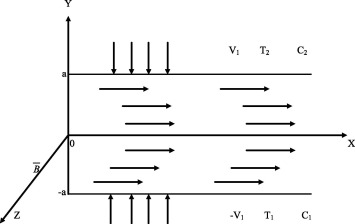Figure 1. The schematic diagram of fluid flow through expanding or contracting walls.

The governing equations of the micropolar fluid flow, heat and mass transfer in the presence of buoyancy forces and magnetic field are  and 

 $\nabla \cdot {\overline {q}}=0$
(1)

 $\rho \left[{\frac {\partial {\overline {q}}}{\partial t}}+\right.$$\left.({\overline {q}}.\nabla ){\overline {q}}\right]=$$-\nabla p+k_{1}\nabla \times {\overline {l}}-\left(\mu +\right.$$\left.k_{1}\right)\nabla \times \nabla \times {\overline {q}}-$${\frac {\mu +k_{1}}{k_{2}}}{\overline {q}}+{\overline {J}}\times {\overline {B}}+$${\overline {F_{b}}}$
(2)

 $\rho j\left[{\frac {\partial {\overline {l}}}{\partial t}}+\right.$$\left.({\overline {q}}.\nabla ){\overline {l}}\right]=$$-2k_{1}{\overline {l}}+k_{1}\nabla \times {\overline {q}}-\gamma \nabla \times \nabla \times {\overline {l}}$
(3)

 $\rho c\left[{\frac {\partial T}{\partial t}}+({\overline {q}}.\nabla )T\right]=$$k{\nabla }^{2}T+2\mu D:D+{\frac {k_{1}}{2}}{\left(curl({\overline {q}})-2{\overline {l}}\right)}^{2}+$$\gamma \nabla {\overline {l}}:\nabla {\overline {l}}+{\frac {\mu +k_{1}}{k_{2}}}{\left|{\overline {q}}\right|}^{2}+$${\frac {{\left|{\overline {J}}\right|}^{2}}{\sigma }}+{\frac {\rho D_{1}k_{T}}{c_{s}}}{\nabla }^{2}C$
(4)

 $\left[{\frac {\partial C}{\partial t}}+({\overline {q}}.\nabla )C\right]=$$D_{1}{\nabla }^{2}C-k_{3}(C-C_{1})+{\frac {D_{1}k_{T}}{T_{m}}}{\nabla }^{2}T$
(5)

where ${\textstyle {\overline {F_{b}}}}$ is the buoyancy force and it is defined as ${\textstyle \left(\rho g{\beta }_{T}(T-T_{1})+\rho g{\beta }_{C}(C-C_{1})\right){\hat {i}}}$.

Neglecting the displacement currents, the Maxwell equations and the generalized Ohm’s law are 

 $\nabla .{\overline {B}}=0{\mbox{,}}\quad \nabla \times {\overline {B}}=$${\mu }^{'}{\overline {J}}{\mbox{,}}\quad \nabla \times {\overline {E}}=$${\frac {\partial {\overline {B}}}{\partial t}}{\mbox{,}}$
 ${\overline {J}}=\sigma ({\overline {E}}+{\overline {q}}\times {\overline {B}})-$${\frac {\beta e}{B_{0}}}\left({\overline {J}}\times {\overline {B}}\right)+$${\frac {\beta e\beta i}{B_{0}^{2}}}\left({\overline {J}}\times {\overline {B}}\right)\times {\overline {B}}$
(6)

where ${\textstyle {\overline {B}}=B_{0}{\hat {k}}+{\overline {b}}{\mbox{,}}{\overline {b}}}$ is induced magnetic field. Assume that the induced magnetic field is negligible compared to the applied magnetic field so that magnetic Reynolds number is small, the electric field is zero and magnetic permeability is constant throughout the flow field.

The velocity and microrotation components are

 ${\overline {q}}\quad =u{\hat {i}}+v{\hat {j}}\quad {\mbox{and}}\quad {\overline {l}}=$$N{\hat {k}}{\mbox{.}}$
(7)

Following Ojjela and Naresh Kumar  the velocity, microrotation, temperature and concentration are,

 $u(x{\mbox{,}}\lambda {\mbox{,}}t)=-{\frac {\upsilon x}{a^{2}}}F^{/}(\lambda {\mbox{,}}t){\mbox{,}}\quad v(x{\mbox{,}}\lambda {\mbox{,}}t)=$${\frac {\upsilon }{a}}F(\lambda {\mbox{,}}t){\mbox{,}}\quad N(x{\mbox{,}}\lambda {\mbox{,}}t)=$${\frac {\upsilon x}{a^{3}}}G(\lambda {\mbox{,}}t)$
 $T(x{\mbox{,}}\lambda {\mbox{,}}t)=T_{1}+{\frac {\left(\mu +k_{1}\right)V_{1}}{\rho ac}}\left[{\varphi }_{1}(\lambda )+\right.$$\left.{\left({\frac {x}{a}}\right)}^{2}{\varphi }_{2}(\lambda )\right]\quad {\mbox{and}}$

 $C(x{\mbox{,}}\lambda {\mbox{,}}t)=C_{1}+{\frac {\overset {\cdot }{n_{A}}}{a\upsilon }}\left[g_{1}(\lambda )+\right.$$\left.{\left({\frac {x}{a}}\right)}^{2}g_{2}(\lambda )\right]$
(8)

where ${\textstyle \lambda ={\frac {y}{h}}}$ and F  (${\textstyle \lambda }$t  ), ${\textstyle G(\lambda {\mbox{,}}t){\mbox{,}}{\phi }_{1}(\lambda ){\mbox{,}}{\phi }_{2}(\lambda ){\mbox{,}}g_{1}(\lambda )}$ and ${\textstyle g_{2}(\lambda )}$ are to be determined.

The boundary conditions for the velocity, microrotation, temperature and concentration are

 ${\begin{array}{rl}&u(x{\mbox{,}}\lambda {\mbox{,}}t)=0{\mbox{,}}v(x{\mbox{,}}\lambda {\mbox{,}}t)=-V_{1}{\mbox{,}}N(x{\mbox{,}}\lambda {\mbox{,}}t)=0{\mbox{,}}\\&T(x{\mbox{,}}\lambda {\mbox{,}}t)=T_{1}{\mbox{,}}C(x{\mbox{,}}\lambda {\mbox{,}}t)=C_{1}\quad {\mbox{at}}\quad \lambda =-1\\&u(x{\mbox{,}}\lambda {\mbox{,}}t)=0{\mbox{,}}v(x{\mbox{,}}\lambda {\mbox{,}}t)=V_{1}{\mbox{,}}N(x{\mbox{,}}\lambda {\mbox{,}}t)=0{\mbox{,}}\\&T(x{\mbox{,}}\lambda {\mbox{,}}t)=T_{2}{\mbox{,}}C(x{\mbox{,}}\lambda {\mbox{,}}t)=C_{2}\quad {\mbox{at}}\quad \lambda =1\end{array}}$
(9)

Substituting (8) in (2), (3), (4) and (5) then we obtain,

 $f^{IV}={\frac {1}{1+R}}(-\eta (3f^{//}+\lambda f^{\left//\right/})+$$Re({ff}^{\left//\right/}-f^{/}f^{//})+{Rg}^{//}+(1+R)D^{-1}f^{//}+$${\frac {{Ha}^{2}\alpha e}{\alpha e^{2}+\beta e^{2}}}f^{//}+$${\frac {Ec\quad Gr}{\zeta \quad Re}}({\phi }_{1}^{/}+{\zeta }^{2}{\phi }_{2}^{/})+$${\frac {Ec\quad Gc}{\zeta \quad Re}}(g_{1}^{/}+{\zeta }^{2}g_{2}^{/}))$
(10)

 $g^{//}=-J_{1}\eta (3g+\lambda g^{/})+J_{1}\quad Re({fg}^{/}-$$f^{/}g)-s_{1}(f^{//}-2g)$
(11)
 ${\varphi }_{1}^{//}=-2{\varphi }_{2}-Re\quad s_{2}g^{2}-Re\quad Pr\left((1+\right.$$\left.R)D^{-1}f^{2}+{\frac {{Ha}^{2}}{\alpha e^{2}+\beta e^{2}}}f^{2}+\right.$$\left.4f^{/^{2}}-f{\varphi }_{1}^{/}\right)$

 $-Pr\quad \eta ({\phi }_{1}+\lambda {\phi }_{1}^{/})-Du(g_{1}^{//}+$$2g_{2})$
(12)

 ${\varphi }_{2}^{//}=-Re\quad Pr\left({\frac {R}{2}}{\left(f^{//}-2g\right)}^{2}+\right.$$\left.{\frac {s_{2}}{Pr}}g^{/^{2}}+(1+R)D^{-1}f^{/^{2}}+{Ha}^{2}f^{/^{2}}+\right.$$\left.f^{//^{2}}+2f^{/}{\varphi }_{2}-f{\varphi }_{2}^{/}\right)-$$Pr\left(3{\phi }_{2}+\lambda {\phi }_{2}^{/}\right)-Du\quad g_{2}^{//}$
(13)

 $g_{1}^{//}=-2g_{2}+Kr\quad g_{1}+Sc\quad Re\quad {fg}_{1}^{/}-$$Sc\quad \eta (g_{1}+\lambda g_{1}^{/})-Sc\quad Sr({\varphi }_{1}^{//}+$$2{\varphi }_{2})$
(14)

 $g_{2}^{//}=Kr\quad g_{2}+Sc\quad Re({fg}_{2}^{/}-2f^{/}g_{2})-$$Sc\quad \eta (3g_{2}+\lambda g_{2}^{/})-Sc\quad Sr{\varphi }_{2}^{//}$
(15)

where prime denotes the differentiation with respect to ${\textstyle \lambda }$ and ${\textstyle f={\frac {F}{Re}}{\mbox{,}}g={\frac {G}{Re}}}$.

The dimensionless form of temperature and concentration from (8) is

 $T^{_{\ast }}={\frac {T-T_{1}}{T_{2}-T_{1}}}=Ec({\phi }_{1}+{\zeta }^{2}{\phi }_{2})$
 $C^{_{\ast }}={\frac {C-C_{1}}{C_{2}-C_{1}}}=Sh(g_{1}+{\zeta }^{2}\quad g_{2})$
(16)

The boundary conditions Eq. (9) in terms of ${\textstyle f{\mbox{,}}g{\mbox{,}}{\varphi }_{1}{\mbox{,}}{\varphi }_{2}{\mbox{,}}g_{1}}$ and ${\textstyle g_{2}}$ are

 $f(-1)=-1{\mbox{,}}\quad f(1)=1{\mbox{,}}$
 $f^{/}(-1)=0{\mbox{,}}\quad f^{/}(1)=0{\mbox{,}}$
 $g(-1)=0{\mbox{,}}\quad g(1)=0$
 ${\varphi }_{1}(-1)=0{\mbox{,}}\quad {\phi }_{1}(1)=1/Ec$
 ${\varphi }_{2}(-1)=0{\mbox{,}}\quad {\phi }_{2}(1)=0{\mbox{,}}$
 $g_{1}(-1)=0{\mbox{,}}\quad g_{1}(1)=1/Sh$
 $g_{2}(-1)=0{\mbox{,}}\quad g_{2}(1)=0$
(17)

## 3. Solution of the problem

The nonlinear Eqs. (10), (11), (12), (13), (14) and (15) are converted into the following system of first order differential equations by the substitution

(${\textstyle f{\mbox{,}}f^{/}{\mbox{,}}f^{//}{\mbox{,}}f^{\left//\right/}{\mbox{,}}g{\mbox{,}}g^{/}{\mbox{,}}{\varphi }_{1}{\mbox{,}}{\varphi }_{1}^{/}{\mbox{,}}{\varphi }_{2}{\mbox{,}}{\varphi }_{2}^{/}{\mbox{,}}g_{1}{\mbox{,}}g_{1}^{/}{\mbox{,}}g_{2}{\mbox{,}}g_{2}^{/}}$) = ${\textstyle \left(x_{1}{\mbox{,}}x_{2}{\mbox{,}}x_{3}{\mbox{,}}x_{4}{\mbox{,}}x_{5}{\mbox{,}}x_{6}{\mbox{,}}x_{7}{\mbox{,}}x_{8}{\mbox{,}}x_{9}{\mbox{,}}x_{10}{\mbox{,}}x_{11}{\mbox{,}}x_{12}{\mbox{,}}x_{13}{\mbox{,}}x_{14}\right)}$

 ${\frac {{dx}_{1}}{d\lambda }}=x_{2}{\mbox{,}}\quad {\frac {{dx}_{2}}{d\lambda }}=$$x_{3}{\mbox{,}}\quad {\frac {{dx}_{3}}{d\lambda }}=x_{4}{\mbox{,}}$
 ${\frac {{dx}_{4}}{d\lambda }}={\frac {R}{1+R}}\left(-s_{1}(x_{3}-\right.$$\left.2x_{5})+J_{1}(x_{1}x_{6}-x_{2}x_{5}-3\eta x_{5}-\lambda \eta x_{6})\right)-$${\frac {Re}{1+R}}(x_{2}x_{3}-x_{1}x_{4})+D^{-1}x_{3}+{\frac {{Ha}^{2}\alpha e}{\left(\alpha e^{2}+\beta e^{2}\right)(1+R)}}x_{3}-$${\frac {\eta }{\left(1+R\right)}}\left(3x_{3}+\lambda x_{4}\right)+$${\frac {Sh\quad Gm}{(1+R)\zeta \quad Re}}(x_{12}+{\zeta }^{2}x_{14})+$${\frac {Ec\quad Gr}{(1+R)\zeta \quad Re}}(x_{8}+{\zeta }^{2}x_{10}){\mbox{,}}$
 ${\frac {{dx}_{5}}{d\lambda }}=x_{6}{\mbox{,}}$
 ${\frac {{dx}_{6}}{d\lambda }}=-s_{1}(x_{3}-2x_{5})+J_{1}(x_{1}x_{6}-$$x_{2}x_{5}-3\eta x_{6}-\lambda \eta x_{7}){\mbox{,}}$
 ${\frac {{dx}_{7}}{d\lambda }}=x_{8}{\mbox{,}}$
 ${\frac {{dx}_{8}}{d\lambda }}=-2x_{9}-{\frac {Re\quad Pr}{1-Du\quad Sc\quad Sr}}\left({\frac {\eta }{Re}}\left(x_{7}+\right.\right.$$\left.\left.\lambda x_{8}\right)+4x_{2}^{2}+(1+R)D^{-1}x_{1}^{2}+\right.$$\left.{\frac {{Ha}^{2}}{\alpha e^{2}+\beta e^{2}}}x_{1}^{2}-x_{1}x_{8}+\right.$$\left.{\frac {s_{2}}{Pr}}x_{5}^{2}\right)-{\frac {Kr\quad Du}{1-Du\quad Sc\quad Sr}}x_{11}-$${\frac {Du\quad Sc}{1-Du\quad Sc\quad Sr}}Re\quad x_{1}x_{12}+$${\frac {Sc\quad Du}{1-Du\quad Sc\quad Sr}}\eta \left(x_{11}+\right.$$\left.\lambda x_{12}\right){\mbox{,}}$
 ${\frac {{dx}_{9}}{d\lambda }}=x_{10}{\mbox{,}}$
 ${\frac {{dx}_{10}}{d\lambda }}=-{\frac {Re\quad Pr}{1-Du\quad Sc\quad Sr}}\left({\frac {\eta }{Re}}\left(3x_{9}+\right.\right.$$\left.\left.\lambda x_{10}\right)+{\frac {R}{2}}{\left(x_{3}-2x_{5}\right)}^{2}+\right.$$\left.x_{3}^{2}+(1+R)D^{-1}x_{2}^{2}+{\frac {{Ha}^{2}}{\alpha e^{2}+\beta e^{2}}}x_{2}^{2}+\right.$$\left.2x_{2}x_{9}-x_{1}x_{10}\right)-{\frac {Du\quad Sc}{1-Du\quad Sc\quad Sr}}Re(x_{1}x_{14}-$$2x_{2}x_{13})+{\frac {Du\quad Sc}{1-Du\quad Sc\quad Sr}}\eta (3x_{13}+$$\lambda x_{14})-{\frac {Kr\quad Du}{1-Du\quad Sc\quad Sr}}x_{13}$
 ${\frac {{dx}_{11}}{d\lambda }}=x_{12}{\mbox{,}}$
 ${\frac {{dx}_{12}}{d\lambda }}=-2x_{13}+{\frac {Sc\quad Sr\quad Re\quad Pr}{1-Du\quad Sc\quad Sr}}\left({\frac {\eta }{Re}}(x_{7}+\right.$$\left.\lambda x_{8})+4x_{2}^{2}+(1+R)D^{-1}x_{1}^{2}+{\frac {{Ha}^{2}}{\alpha e^{2}+\beta e^{2}}}x_{1}^{2}-\right.$$\left.x_{1}x_{8}+{\frac {s_{2}}{Pr}}x_{5}^{2}\right)+{\frac {Kr}{1-Du\quad Sc\quad Sr}}x_{11}+$${\frac {Sc}{1-Du\quad Sc\quad Sr}}Re\quad x_{1}x_{12}-{\frac {Sc}{1-Du\quad Sc\quad Sr}}\eta (x_{11}+$$\lambda x_{12}){\mbox{,}}$
 ${\frac {{dx}_{13}}{d\lambda }}=x_{14}{\mbox{,}}$

 ${\frac {{dx}_{14}}{d\lambda }}={\frac {Sc\quad Sr\quad Re\quad Pr}{1-Du\quad Sc\quad Sr}}\left({\frac {\eta }{Re}}(3x_{9}+\right.$$\left.\lambda x_{10})+x_{3}^{2}+(1+R)D^{-1}x_{2}^{2}+{\frac {{Ha}^{2}}{\alpha e^{2}+\beta e^{2}}}x_{2}^{2}+\right.$$\left.{\frac {s_{2}}{Pr}}x_{6}^{2}+{\frac {R}{2}}{\left(x_{3}-2x_{5}\right)}^{2}+\right.$$\left.2x_{2}x_{9}-x_{1}x_{10}\right)+{\frac {Kr}{1-Du\quad Sc\quad Sr}}x_{13}+$${\frac {Sc}{1-Du\quad Sc\quad Sr}}Re(x_{1}x_{14}-2x_{2}x_{13})-$${\frac {Sc}{1-Du\quad Sc\quad Sr}}\eta (3x_{13}+\lambda x_{14})$
(18)

The boundary conditions in terms of ${\textstyle x_{1}{\mbox{,}}x_{2}{\mbox{,}}x_{3}{\mbox{,}}x_{4}x_{5}{\mbox{,}}x_{6}{\mbox{,}}x_{7}{\mbox{,}}x_{8}{\mbox{,}}x_{9}{\mbox{,}}x_{10}{\mbox{,}}x_{11}{\mbox{,}}x_{12}{\mbox{,}}x_{13}{\mbox{,}}x_{14}}$ are

 ${\begin{array}{cc}&x_{1}(-1)=-1{\mbox{,}}\quad x_{2}(-1)=0{\mbox{,}}\quad x_{5}(-1)=0{\mbox{,}}\quad x_{7}(-1)=0{\mbox{,}}\quad x_{9}(-1)=0{\mbox{,}}\quad x_{11}(-1)=0{\mbox{,}}\quad x_{13}(-1)=0{\mbox{,}}\\&x_{1}(1)=1{\mbox{,}}\quad x_{2}(1)=0{\mbox{,}}\quad x_{5}(1)=0{\mbox{,}}\quad x_{7}(1)=1/Ec{\mbox{,}}\quad x_{9}(1)=0{\mbox{,}}\quad x_{11}(1)=1/Sh{\mbox{,}}\quad x_{13}(1)=0\end{array}}$
(19)

The system of Eq. (18) is solved numerically subject to the boundary conditions (19) using the quasilinearization method given by Bellman and Kalaba .

Let (${\textstyle x_{i}^{r}}$, i = 1, 2, … , 14) be an approximate current solution and ${\textstyle (x_{i}^{r+1}}$, i = 1, 2, … , 14) be an improved solution of (18). Using Taylor’s series expansion about the current solution by neglecting the second and higher order derivative terms, the coupled first order system (18) is linearized as follows:

 ${\frac {{dx}_{1}^{r+1}}{d\lambda }}\quad =x_{2}^{r+1}{\mbox{,}}\quad {\frac {{dx}_{2}^{r+1}}{d\lambda }}\quad =$$x_{3}^{r+1}{\mbox{,}}\quad {\frac {{dx}_{3}^{r+1}}{d\lambda }}\quad =$$\quad x_{4}^{r+1}{\mbox{,}}$
 ${\frac {{dx}_{4}^{r+1}}{d\lambda }}={\frac {Re}{1+R}}(x_{1}^{r+1}x_{4}^{r}+$$x_{4}^{r+1}x_{1}^{r}-x_{2}^{r+1}x_{3}^{r}-x_{2}^{r}x_{3}^{r+1})+D^{-1}x_{3}^{r+1}+$${\frac {{Ha}^{2}\quad \alpha e\quad x_{3}^{r+1}}{\left(1+R\right)\left(\alpha e^{2}+\beta e^{2}\right)}}+$${\frac {R}{1+R}}(-s_{1}(x_{3}^{r+1}-2x_{5}^{r+1})+J_{1}(x_{1}^{r+1}x_{6}^{r}+$$x_{1}^{r}x_{6}^{r+1}-x_{2}^{r+1}x_{5}^{r}-x_{5}^{r+1}x_{2}^{r}-3\eta x_{5}^{r+1}-$$\lambda \eta x_{6}^{r+1}))+{\frac {E\quad Gr}{(1+R)\zeta \quad Re}}\left(x_{8}^{r+1}+\right.$$\left.{\zeta }^{2}x_{10}^{r+1}\right)+{\frac {Sh\quad Gm}{(1+R)\zeta \quad Re}}\left(x_{12}^{r+1}+\right.$$\left.{\zeta }^{2}x_{14}^{r+1}\right)-{\frac {Re}{1+R}}\left(-\right.$$\left.x_{2}^{r}x_{3}^{r}+x_{1}^{r}x_{4}^{r}\right)-{\frac {J_{1}}{1+R}}(x_{1}^{r}x_{6}^{r}-$$x_{2}^{r}x_{5}^{r})-{\frac {\eta }{\left(1+R\right)}}\left(3x_{3}^{r+1}+\right.$$\left.\lambda x_{4}^{r+1}\right){\mbox{,}}$
 ${\frac {{dx}_{5}^{r+1}}{d\lambda }}\quad =\quad x_{6}^{r+1}{\mbox{,}}$
 ${\frac {{dx}_{6}^{r+1}}{d\lambda }}=-s_{1}(x_{3}^{r+1}-2x_{5}^{r+1})+$$J_{1}(x_{1}^{r+1}x_{6}^{r}+x_{1}^{r}x_{6}^{r+1}-x_{2}^{r+1}x_{5}^{r}-x_{5}^{r+1}x_{2}^{r}-$$3\eta x_{5}^{r+1}-\lambda \eta x_{6}^{r+1})-J_{1}(x_{1}^{r}x_{6}^{r}-$$x_{2}^{r}x_{5}^{r}){\mbox{,}}\quad {\frac {{dx}_{7}^{r+1}}{d\lambda }}=$$x_{8}^{r+1}$
 ${\frac {{dx}_{8}^{r+1}}{d\lambda }}=-2x_{9}^{r+1}-{\frac {Re\quad Pr}{1-Du\quad Sc\quad Sr}}\left({\frac {\eta }{Re}}\left(x_{7}^{r+1}+\right.\right.$$\left.\left.\lambda x_{8}^{r+1}\right)+8x_{2}^{r}x_{2}^{r+1}+\right.$$\left.2(1+R)D^{-1}x_{1}^{r}x_{1}^{r+1}+{\frac {2{Ha}^{2}}{\alpha e^{2}+\beta e^{2}}}x_{1}^{r}x_{1}^{r+1}-\right.$$\left.x_{1}^{r}x_{8}^{r+1}-x_{8}^{r}x_{1}^{r+1}+{\frac {2s_{2}}{Pr}}x_{5}^{r}x_{5}^{r+1}\right)-$${\frac {Kr\quad Du}{1-Du\quad Sc\quad Sr}}x_{11}^{r+1}+$${\frac {Sc\quad Du}{1-Du\quad Sc\quad Sr}}\eta \left(x_{11}^{r+1}+\right.$$\left.\lambda x_{12}^{r+1}\right)-{\frac {Du\quad Sc}{1-Du\quad Sc\quad Sr}}Re\left(x_{1}^{r}x_{12}^{r+1}+\right.$$\left.x_{12}^{r}x_{1}^{r+1}-x_{1}^{r}x_{12}^{r}\right)+{\frac {Re\quad Pr}{1-Du\quad Sc\quad Sr}}\left(4x_{2}^{r}x_{2}^{r}+\right.$$\left.(1+R)D^{-1}x_{1}^{r}x_{1}^{r}+{\frac {{Ha}^{2}}{\alpha e^{2}+\beta e^{2}}}x_{1}^{r}x_{1}^{r}-\right.$$\left.x_{1}^{r}x_{8}^{r}+{\frac {s_{2}}{Pr}}x_{5}^{r}x_{5}^{r}\right){\mbox{,}}\quad {\frac {{dx}_{9}^{r+1}}{d\lambda }}=$$x_{10}^{r+1}{\mbox{,}}$
 ${\frac {{dx}_{10}^{r+1}}{d\lambda }}=-{\frac {Re\quad Pr}{1-Du\quad Sc\quad Sr}}\left({\frac {\eta }{Re}}\left(3x_{9}^{r+1}+\right.\right.$$\left.\left.\lambda x_{10}^{r+1}\right)+{\frac {R}{2}}(2x_{3}^{r}x_{3}^{r+1}+\right.$$\left.8x_{5}^{r}x_{5}^{r+1}-4x_{3}^{r}x_{5}^{r+1}-4x_{5}^{r}x_{3}^{r+1})+\right.$$\left.2x_{3}^{r}x_{3}^{r+1}+2(1+R)D^{-1}x_{2}^{r}x_{2}^{r+1}+\right.$$\left.{\frac {2\quad {Ha}^{2}}{\alpha e^{2}+\beta e^{2}}}x_{2}^{r}x_{2}^{r+1}+\right.$$\left.2x_{2}^{r}x_{9}^{r+1}+2x_{2}^{r+1}x_{9}^{r}-x_{1}^{r}x_{10}^{r+1}-\right.$$\left.x_{1}^{r+1}x_{10}^{r}\right)+{\frac {Du\quad Sc}{1-Du\quad Sc\quad Sr}}\eta (3x_{13}^{r+1}+$$\lambda x_{14}^{r+1})-{\frac {Du\quad Sc}{1-Du\quad Sc\quad Sr}}Re(x_{1}^{r}x_{14}^{r+1}+$$x_{1}^{r+1}x_{14}^{r}-2x_{2}^{r}x_{13}^{r+1}-2x_{2}^{r+1}x_{13}^{r}-$$x_{1}^{r}x_{14}^{r}+2x_{2}^{r}x_{13}^{r})+{\frac {Re\quad Pr}{1-Du\quad Sc\quad Sr}}\left({\frac {R}{2}}(x_{3}^{r}x_{3}^{r}+\right.$$\left.4x_{5}^{r}x_{5}^{r}-4x_{3}^{r}x_{5}^{r})+x_{3}^{r}x_{3}^{r}+(1+R)D^{-1}x_{2}^{r}x_{2}^{r}+\right.$$\left.{\frac {{Ha}^{2}}{\alpha e^{2}+\beta e^{2}}}x_{2}^{r}x_{2}^{r}+\right.$$\left.2x_{2}^{r}x_{9}^{r}-x_{1}^{r}x_{10}^{r}\right)-{\frac {Kr\quad Du}{1-Du\quad Sc\quad Sr}}x_{13}^{r+1}{\mbox{,}}$
 ${\frac {{dx}_{11}^{r+1}}{d\lambda }}=x_{12}^{r+1}{\mbox{,}}$
 ${\frac {{dx}_{12}^{r+1}}{d\lambda }}=-2x_{13}^{r+1}+{\frac {Sc\quad Sr\quad Re\quad Pr}{1-Du\quad Sc\quad Sr}}\left({\frac {\eta }{Re}}(x_{7}^{r+1}+\right.$$\left.\lambda x_{8}^{r+1})+8x_{2}^{r}x_{2}^{r+1}+2(1+R)D^{-1}x_{1}^{r}x_{1}^{r+1}+\right.$$\left.{\frac {2{Ha}^{2}}{\alpha e^{2}+\beta e^{2}}}x_{1}^{r}x_{1}^{r+1}-\right.$$\left.x_{1}^{r}x_{8}^{r+1}-x_{1}^{r+1}x_{8}^{r}+{\frac {2s_{2}}{Pr}}x_{5}^{r}x_{5}^{r+1}\right)-$${\frac {Sc}{1-Du\quad Sc\quad Sr}}\eta (x_{11}^{r+1}+$$\lambda x_{12}^{r+1})+{\frac {Kr}{1-Du\quad Sc\quad Sr}}x_{11}^{r+1}+$${\frac {Sc}{1-Du\quad Sc\quad Sr}}Re(x_{1}^{r}x_{12}^{r+1}+$$x_{1}^{r+1}x_{12}^{r}-x_{1}^{r}x_{12}^{r})-{\frac {Sc\quad Sr\quad Re\quad Pr}{1-Du\quad Sc\quad Sr}}\left(4x_{2}^{r}x_{2}^{r}+\right.$$\left.(1+R)D^{-1}x_{1}^{r}x_{1}^{r}+{\frac {{Ha}^{2}}{\alpha e^{2}+\beta e^{2}}}x_{1}^{r}x_{1}^{r}-\right.$$\left.x_{1}^{r}x_{8}^{r}+{\frac {s_{2}}{Pr}}x_{5}^{r}x_{5}^{r}\right){\mbox{,}}$
 ${\frac {{dx}_{13}^{r+1}}{d\lambda }}=x_{14}^{r+1}{\mbox{,}}$

 ${\frac {{dx}_{14}^{r+1}}{d\lambda }}={\frac {Sc\quad Sr\quad Re\quad Pr}{1-Du\quad Sc\quad Sr}}\left({\frac {\eta }{Re}}(3x_{9}^{r+1}+\right.$$\left.\lambda x_{10}^{r+1})+2x_{3}^{r}x_{3}^{r}+2(1+R)D^{-1}x_{2}^{r}x_{2}^{r+1}+\right.$$\left.{\frac {2{Ha}^{2}}{\alpha e^{2}+\beta e^{2}}}x_{2}^{r}x_{2}^{r+1}+\right.$$\left.{\frac {2s_{2}}{Pr}}x_{6}^{r}x_{6}^{r+1}+{\frac {R}{2}}(2x_{3}^{r}x_{3}^{r+1}+\right.$$\left.8x_{5}^{r}x_{5}^{r+1}-4x_{3}^{r}x_{5}^{r+1}-4x_{5}^{r}x_{3}^{r+1})+\right.$$\left.2x_{2}^{r}x_{9}^{r+1}+2x_{2}^{r+1}x_{9}^{r}-x_{1}^{r}x_{10}^{r+1}-\right.$$\left.x_{1}^{r+1}x_{10}^{r}\right)+{\frac {Kr}{1-Du\quad Sc\quad Sr}}x_{13}^{r+1}+$${\frac {Sc}{1-Du\quad Sc\quad Sr}}Re(x_{1}^{r}x_{14}^{r+1}+$$x_{1}^{r+1}x_{14}^{r}-2x_{2}^{r}x_{13}^{r+1}-2x_{2}^{r+1}x_{13}^{r}-$$x_{1}^{r}x_{14}^{r}+2x_{2}^{r}x_{13}^{r})-{\frac {Sc}{1-Du\quad Sc\quad Sr}}\eta (3x_{13}^{r+1}+$$\lambda x_{14}^{r+1})-{\frac {Sc\quad Sr\quad Re\quad Pr}{1-Du\quad Sc\quad Sr}}(x_{3}^{r}x_{3}^{r}+$$(1+R)D^{-1}x_{2}^{r}x_{2}^{r}+{\frac {{Ha}^{2}}{\alpha e^{2}+\beta e^{2}}}x_{2}^{r}x_{2}^{r}+$${\frac {s_{2}}{Pr}}x_{6}^{r}x_{6}^{r}+{\frac {R}{2}}(x_{3}^{r}x_{3}^{r}+4x_{5}^{r}x_{5}^{r}-$$4x_{3}^{r}x_{5}^{r})+2x_{2}^{r}x_{9}^{r}-x_{1}^{r}x_{10}^{r})$
(20)

To solve for (${\textstyle x_{i}^{r+1}}$, i = 1, 2, … , 14), the solution to seven separate initial value problems, are denoted by ${\textstyle x_{i}^{h1}(\lambda ){\mbox{,}}x_{i}^{h2}(\lambda ){\mbox{,}}x_{i}^{h3}(\lambda ){\mbox{,}}x_{i}^{h4}(\lambda ){\mbox{,}}x_{i}^{h5}(\lambda ){\mbox{,}}x_{i}^{h6}(\lambda ){\mbox{,}}x_{i}^{h7}(\lambda )}$ (which are the solutions of the homogeneous system corresponding to (20)) and ${\textstyle x_{i}^{p1}(\lambda )}$ (which is the particular solution of (20)), with the following initial conditions are obtained by using the 4th order Runge–Kutta method.

 ${\begin{array}{rl}&x_{3}^{h1}(0)=1{\mbox{,}}\quad x_{i}^{h1}(0)=0\quad {\mbox{for}}\quad i\quad \not =\quad 3{\mbox{,}}\\&x_{4}^{h2}(0)=1{\mbox{,}}\quad x_{i}^{h2}(0)=0\quad {\mbox{for}}\quad i\quad \quad \not =\quad 4{\mbox{,}}\\&x_{6}^{h3}(0)=1{\mbox{,}}\quad x_{i}^{h3}(0)=0\quad {\mbox{for}}\quad i\quad \quad \not =\quad 6{\mbox{,}}\\&x_{8}^{h4}(0)=1{\mbox{,}}\quad x_{i}^{h4}(0)=0\quad {\mbox{for}}\quad i\quad \quad \not =\quad 8{\mbox{,}}\\&x_{10}^{h5}(0)=1{\mbox{,}}\quad x_{i}^{h5}(0)=0\quad {\mbox{for}}\quad i\quad \quad \not =\quad 10{\mbox{,}}\\&x_{12}^{h6}(0)=1{\mbox{,}}\quad x_{i}^{h6}(0)=0\quad {\mbox{for}}\quad i\quad \not =\quad 12{\mbox{,}}\\&x_{14}^{h7}(0)=1{\mbox{,}}\quad x_{i}^{h7}(0)=0\quad {\mbox{for}}\quad i\quad \not =\quad 14{\mbox{,}}\\&x_{1}^{p1}(0)=-1{\mbox{,}}\\&x_{2}^{p1}(0)=x_{3}^{p1}(0)=x_{4}^{p1}(0)=x_{5}^{p1}(0)=0\end{array}}$
 $x_{6}^{p1}(0)=x_{7}^{p1}(0)=x_{8}^{p1}(0)=x_{9}^{p1}(0)=x_{10}^{p1}(0)=$$x_{11}^{p1}(0)=x_{12}^{p1}(0)=x_{13}^{p1}(0)=x_{14}^{p1}(0)=$$0$
(21)

By using the principle of superposition, the general solution can be written as

 $x_{i}^{n+1}(\lambda )=C_{1}x_{i}^{h1}(\lambda )+C_{2}x_{i}^{h2}(\lambda )+$$C_{3}x_{i}^{h3}(\lambda )+C_{4}x_{i}^{h4}(\lambda )+C_{5}x_{i}^{h5}(\lambda )+$$C_{6}x_{i}^{h6}(\lambda )+C_{7}x_{i}^{h7}(\lambda )+x_{i}^{p1}(\lambda )$
(22)

where ${\textstyle C_{1}{\mbox{,}}C_{2}{\mbox{,}}C_{3}{\mbox{,}}C_{4}{\mbox{,}}C_{5}{\mbox{,}}C_{6}}$ and ${\textstyle C_{7}}$ are the unknown constants and are determined by considering the boundary conditions at ${\textstyle \lambda =1}$. This solution ${\textstyle (x_{i}^{r+1}}$, i = 1, 2, … , 14) is then compared with solution at the previous step (${\textstyle x_{i}^{r}}$, i = 1, 2, … , 14) and further iteration is performed if the convergence has not been achieved.

## 4. Results and discussion

A numerical solution for the system of nonlinear differential equations Eq. (18) subject to the boundary conditions Eq. (19) is obtained by the method of quasilinearization. The effects of various parameters such as Soret number Sr, Dufour number Du, chemical reaction parameter Kr, inverse Darcy’s parameter D−1, Hall parameter ${\textstyle \beta e}$ and ion slip parameter ${\textstyle \beta i}$ on nondimensional velocity components, temperature distribution, microrotation and concentration are discussed through graphs in the domain [−1, 1].

The effect of Sr on temperature and concentration is presented in Fig. 2. From this it is evident that as Sr increases the concentration is also increasing, whereas the temperature distribution is decreasing. This is because of the mass flux created by the temperature gradient is inversely proportional to the mean temperature, this causes the loss of temperature of the fluid and the concentration of the fluid increases due to the thermal diffusion rate is increasing with the suction/injection velocity. Fig. 3 displays the change in the temperature distribution and concentration for different values of Du. From this it is observed that when Du increases, the temperature distribution and concentration are decreasing toward the upper wall. This is due to the fact that the energy flux created by the concentration gradient is inversely proportional to the suction/injection velocity. Fig. 4 describes the behavior of the temperature distribution and concentration for various values of Kr. As Kr   increases the temperature distribution of the fluid also increases, whereas the concentration decreases toward the upper wall. It means that increase in the chemical reaction rate produces a decrease in the species concentration. This causes the concentration buoyancy effects to decrease as chemical reaction increases. The effect of ion slip parameter ${\textstyle \beta i}$ on velocity components, microrotation, temperature distribution and concentration is presented in Fig. 5. From this it is noticed that as ${\textstyle \beta i}$ increases the temperature is increasing, whereas concentration is decreasing toward the upper wall and the microrotation decreases in the region ${\textstyle -1<\lambda <0}$ and increases in ${\textstyle 0<\lambda <1}$ whereas the radial velocity follows the opposite trend of microrotation. However, the axial velocity attains the maximum at the center of the walls. This is due to decrease in the effective conductivity which reduces the damping force on the flow field. Fig. 6 displays the effect of Hall parameter ${\textstyle \beta e}$ on velocity components, microrotation, temperature and concentration. As ${\textstyle \beta e}$ increases the profiles of velocity components, microrotation, temperature and concentration follow the similar trend of ${\textstyle \beta i}$. This is because of the velocity of the fluid increases with current density. The variation of the velocity components, microrotation, temperature distribution and concentration for different values of D−1 is shown in Fig. 7. From this one can analyze that the temperature and concentration are increasing with D−1 and the radial velocity decreases up to the center of the channel, and then increases whereas the microrotation follows the opposite trend of radial velocity. However, As D−1 increases the axial velocity decreases at the center of the walls and increasing near the walls due to the resistance offered by the porosity of the medium is more than the resistance due to the magnetic lines of force.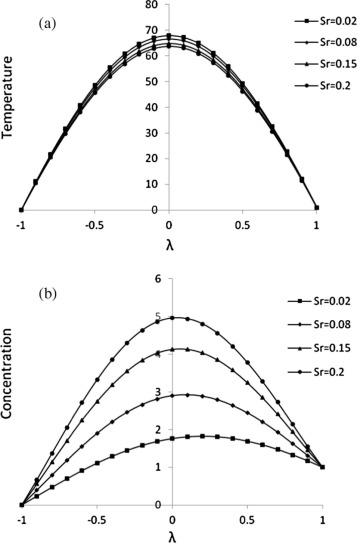Figure 2. Effect of Sr on (a) temperature and (b) concentration for Kr = 0.2, Gr = 5, Gm = 5, Re = 2, Du = 0.2, Sc = 0.8, Pr = 0.2, R = 10, J1 = 0.2, s1 = 2, s2 = 2, Ha = 2, D−1 = 2, ${\textstyle \beta }$e = 0.2, ${\textstyle \beta }$i = 0.2, ${\textstyle \eta }$ = 2.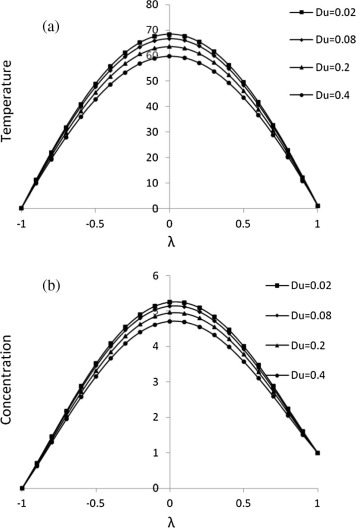Figure 3. Effect of Du on (a) temperature and (b) concentration for Kr = 0.2, Gr = 5, Gm = 5, Re = 2, Sr = 0.2, Sc = 0.8, Pr = 0.2, R = 10, J1 = 0.2, s1 = 2, s2 = 2, Ha = 2, D−1 = 2, ${\textstyle \beta }$e = 0.2, ${\textstyle \beta }$i = 0.2, ${\textstyle \eta }$ = 2.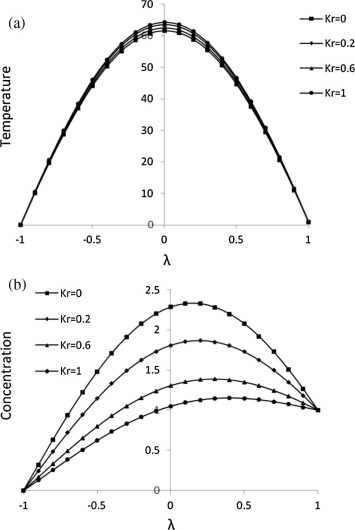Figure 4. Effect of Kr on (a) temperature and (b) concentration for Du = 2, Gr = 5, Gm = 5, Re = 2, Sr = 0.02, Sc = 0.8, Pr = 0.2, R = 10, J1 = 0.2, s1 = 2, s2 = 2, Ha = 2, D−1 = 2, ${\textstyle \beta }$e = 0.2, ${\textstyle \beta }$i = 0.2, ${\textstyle \eta }$ = 2.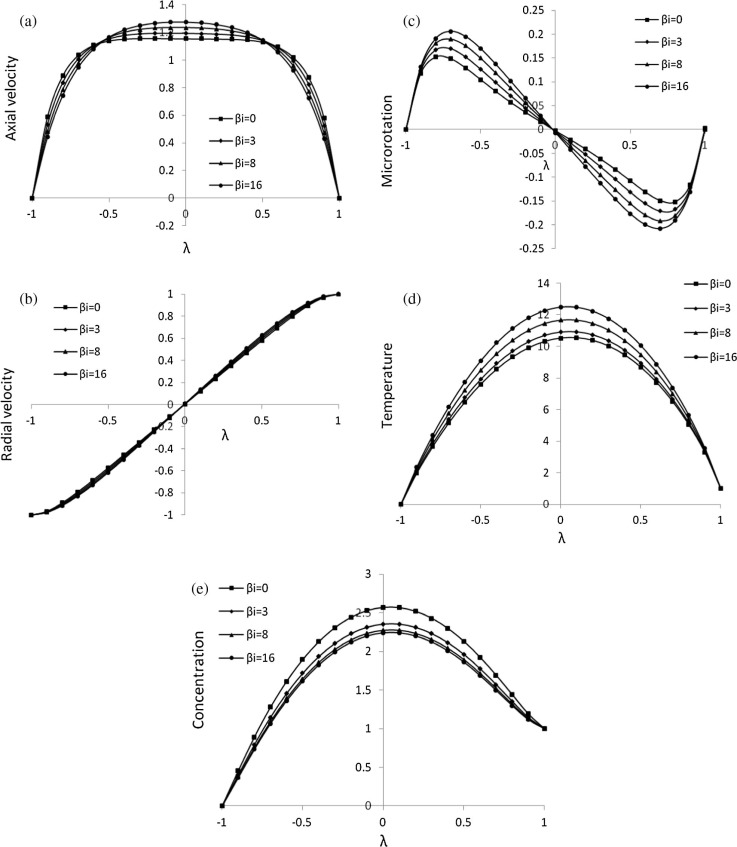Figure 5. Effect of ${\textstyle \beta }$i on (a) axial velocity, (b) radial velocity, (c) microrotation, (d) temperature and (e) concentration for Du = 2, Gr = 5, Gm = 5, Re = 2, Sr = 0.2, Sc = 0.8, Pr = 0.2, R = 10, J1 = 0.2, s1 = 2, s2 = 2, Ha = 2, D−1 = 2, Kr = 2, ${\textstyle \beta }$e = 0.2, ${\textstyle \eta }$ = 0.2.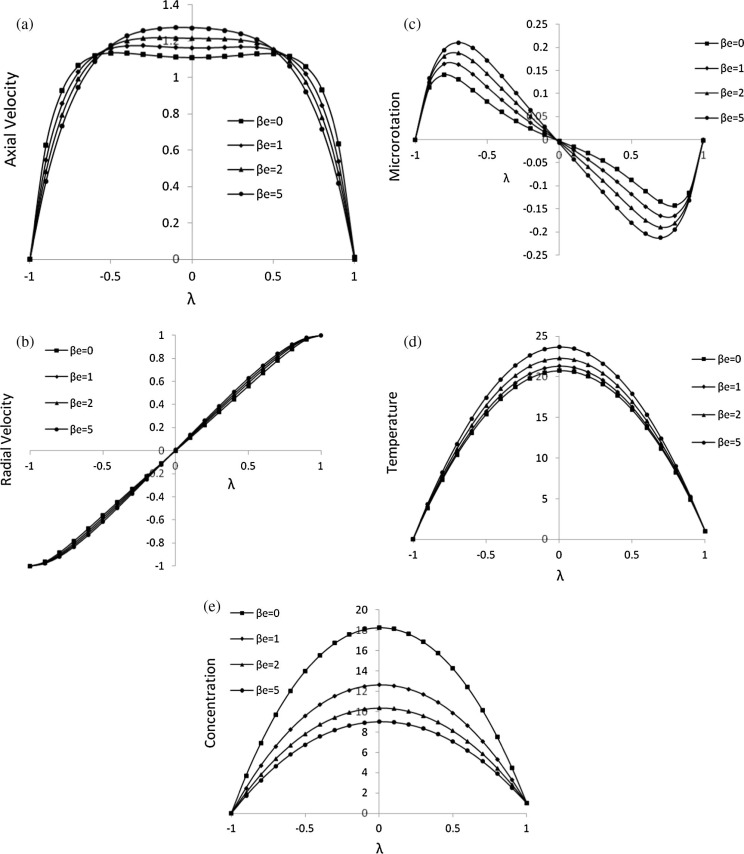Figure 6. Effect of ${\textstyle \beta }$e on (a) axial velocity, (b) radial velocity, (c) microrotation, (d) temperature and (e) concentration for Du = 0.02, Gr = 5, Gm = 5, Re = 2, Sr = 0.2, Sc = 0.8, Pr = 0.2, R = 10, J1 = 0.2, s1 = 4, s2 = 2, Ha = 2, D−1 = 2, Kr = 2, ${\textstyle \beta }$i = 5, ${\textstyle \eta }$ = 0.2.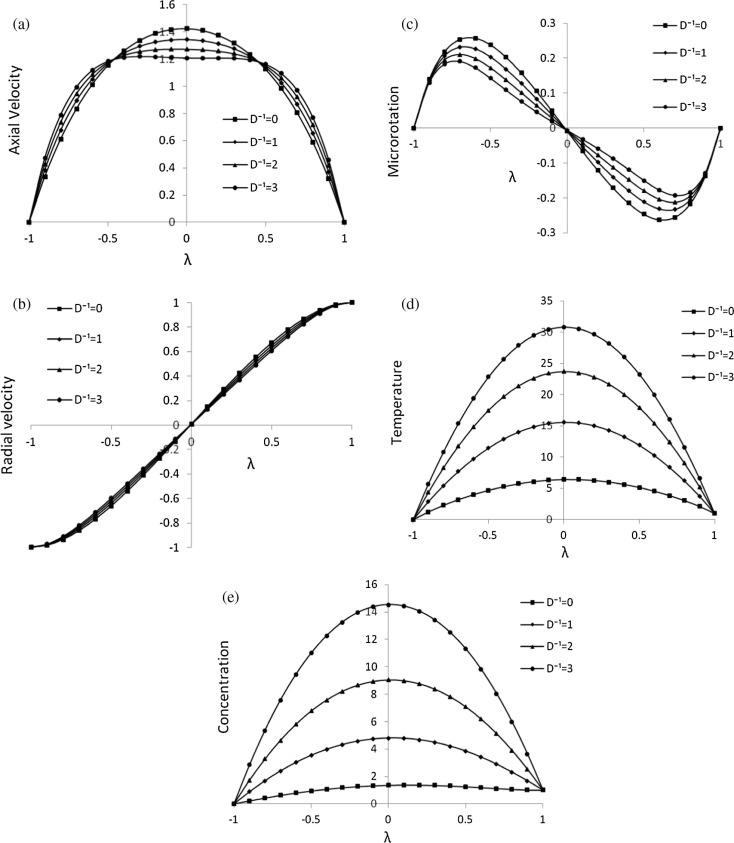Figure 7. Effect of D−1 on (a) axial velocity, (b) radial velocity, (c) microrotation, (d) temperature and (e) concentration for Du = 0.02, Gr = 5, Gm = 5, Re = 2, Sr = 0.2, Sc = 0.8, Pr = 0.2, R = 10, J1 = 0.2, s1 = 2, s2 = 2, Ha = 2, ${\textstyle \beta }$i = 0.2, Kr = 2, ${\textstyle \beta }$e = 5, ${\textstyle \eta }$ = 0.2.

The numerical values of axial velocity with ${\textstyle {\alpha }_{2}=0.5}$ and Re = 5 for Newtonian fluid case are presented in Table 1. It is observed that the results are showing excellent agreement with Majdalani et al. , Asghar et al.  and Boutros et al. .

Table 1. The numerical values of axial velocity with present and existing results for ${\textstyle \eta }$ = 0.5 and Re = 5 (Newtonian case).
${\textstyle \lambda }$ Present Majdalani et al.  Asghar et al.  Boutros et al. 
0 1.53785 1.536002 1.559474 1.556324
0.05 1.53344 1.531846 1.554822 1.551780
0.1 1.52025 1.519377 1.540888 1.538164
0.15 1.49834 1.498596 1.517743 1.515522
0.2 1.46782 1.469505 1.485503 1.483935
0.25 1.42883 1.432114 1.444331 1.443517
0.3 1.38158 1.386445 1.394435 1.394421
0.35 1.32627 1.332539 1.336071 1.336839
0.4 1.26318 1.270464 1.269540 1.271006
0.45 1.19258 1.200325 1.195188 1.197207
0.5 1.11478 1.122275 1.113403 1.115778
0.55 1.03011 1.036527 1.024617 1.027110
0.6 0.93888 0.943364 0.929302 0.931656
0.65 0.84142 0.843156 0.827971 0.829933
0.7 0.73802 0.736373 0.721170 0.722523
0.75 0.62892 0.623597 0.609480 0.610078
0.8 0.51432 0.505538 0.493513 0.493322
0.85 0.39429 0.383052 0.373909 0.373046
0.9 0.26877 0.257149 0.251330 0.250109
0.95 0.13751 0.129010 0.126461 0.125435
1 0.000000 0.000000 0.000000 0.000000

## 5. Conclusions

The influence of Hall and ion slip currents on the free convective flow of chemically reacting micropolar fluid in a porous expanding or contracting walls with Soret and Dufour effects is considered. The numerical solution of the reduced governing equations is obtained by the method of quasilinearization. It is observed that.

• The temperature distribution of the fluid is decreased with the increase of Sr and Du and the concentration of the fluid is enhanced with Sr whereas it is decreased as Du increases.
• The velocity components, microrotation, temperature and concentration of the fluid have the similar effects for Hall and ion slip parameters.
• The concentration of the fluid is decreased whereas the temperature is enhanced with Kr.
• D−1 exhibits the similar effect for temperature and concentration of the fluid.
• The present results are compared with previously published work ,  and  and found that the axial velocity values are showing a remarkable agreement.

These results have possible applications in engineering and applied sciences such as the regression of the burning surface in solid rocket motors, paper manufacturing, irrigation, and transport of biological fluids through expanding or contracting vessels.

## Acknowledgments

The authors are thankful to the editor and referees for their valuable suggestions to improve the version of the paper. Also, one of the authors (N. N. K) gratefully acknowledges the Defence Research and Development Organization (DRDO), Government of India for the financial support as a Senior Research Fellowship (DIAT/F/REG(G)/1613).

### Document informationPublished on 12/04/17

Licence: Other

### Document Score0

Views 19
Recommendations 0